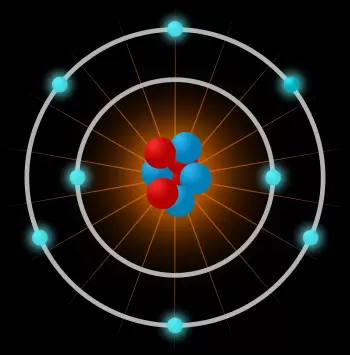# Structure of the Atom, Atomic Parts and ParticlesAn atom is the smallest constituent unit of ordinary matter that has the properties of a chemical element. The atom is composed of:

The main difference between protons, neutrons, and electrons is their electrical charge. Electrons have a negative electric charge, protons have a positive charge, and neutrons are particles with a neutral electric charge.

Neutral atoms have the same number of electrons as protons since both subparticles have the same electrical charge.

An atom is made up of a very dense central nucleus, with the nucleons close together, and electrons that move around the nucleus at a relatively great distance.

## Structure of the Nucleus of the Atom

The atomic nucleus is the central part of the atom that is composed of nucleons joined together by very strong bonds. A nucleon can be a proton or a neutron.

Because protons have a positive electrical charge and neutrons have a neutral charge, the natural tendency is to separate. The bonds that hold the nucleons together exert a nuclear force much stronger than the electromagnetic force at short distances.

The strength of these nuclear bonds makes it possible to overcome the electrical repulsion between the protons.

### What Is the Mass Number of an Atom?

The mass number of an atom is the number of nucleons in its atomic nucleus, that is, the sum of protons and neutrons.

The volume of the nucleus is roughly proportional to the total number of nucleons, the mass number.

### What Does the Atomic Number Represent?

The atomic number is the number of protons that an atom has and is represented by Z.

Atoms are classified in the periodic table of the elements according to atomic number.

### Isotopes: Atoms with a Different Nucleus Composition

The atoms of a chemical element must have the same number of protons, but they can have a different number of neutrons. Atoms of the same element with different numbers of neutrons are called isotopes.

Isotopes can be found naturally but can also be manipulated. For example, uranium enrichment consists of increasing the number of neutrons in a uranium atom to make it more unstable and favor fission reactions.

## What Is the Electron Cloud?

The electron is a stable elementary particle with the smallest negative charge that exists in nature. This charge is called an elemental charge, since any separable electrical charge is made up of an integer of them.

The electrons have a negative electric charge, therefore they are attracted by the protons, of positive sign in the atom through the electromagnetic force. This force increases by reducing the distance between the electron and the nucleus.

The existence of the electron was discovered by JJ Thomson. From this moment it already appeared in all the atomic models developed in the future.

## Atomic Orbitals: the Probability of Finding an Electron at a Point

Electrons tend to form a certain type of standing wave around the atomic nucleus. Each of these waves is characterized by an atomic orbital, a mathematical function that describes the probability of finding the electron at each point in space.

The electron cloud is the region occupied by these waves, visualized as a negative charge density around the nucleus.

Each orbital corresponds to a possible energy value for electrons. However, an electron can jump to a higher level or a lower level by capturing or emitting energy.

The concept of the atomic orbital was first introduced in Schrödinger's atomic model.

## How Important Is the Composition of an Atom to Nuclear Energy?

The basis of everything related to nuclear energy lies in the nucleus of the atom, since nuclear technology is based on the use of the internal energy contained in the atoms.

Nuclear energy is obtained from the division of the nucleus of an atom (nuclear fission) or from the fusion of two atomic nuclei (nuclear fusion).

When this happens and the structure of the atom changes, a large amount of thermal energy is released.

### Nuclear Reactions: Fission and Fusion of Atoms

The atomic nucleus can be altered by very energetic processes that can cause a change in the structure of the atom. Unstable uranium and plutonium nuclei can undergo decays that can change the number of protons and neutrons by emitting radiation.

A heavy nucleus can fission into lighter nuclei in a nuclear reaction or spontaneously. By means of a sufficient amount of energy, two or more nuclei can fuse into another heavier one, in this case, it would be a nuclear fusion reaction.

Chemical elements that have a low atomic number, nuclei with different numbers of protons and neutrons tend to disintegrate into nuclei with more similar proportions. However, the heavier elements need a higher proportion of neutrons to stabilize the nucleus.

Author:

Published: March 14, 2019
Last review: September 6, 2021